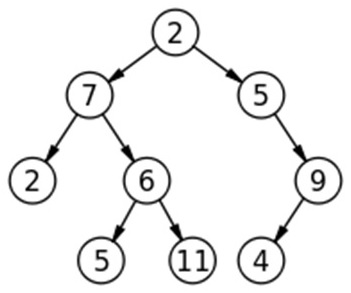Q:

# K distance from root

Given a Binary Tree and a number KPrint all nodes that are at distance K from root (root is considered at distance 0 from itself). Nodes should be printed from left to right. If K is more that height of tree, nothing should be printed.

Example:```    For the above tree:

K=3
Output:
5, 11, 4

K=4
Output:
No output```

The above problem can be solved using level order traversal. For every level traversed, keep decrementing K.

Algorithm

Pre-requisite:

A Queue, input binary tree, input K

```FUNCTION printKdistance(root, k)
1.  Declare a queueqto store tree nodes.
EnQueue(q, root);
EnQueue(q, NULL);
NULL is EnQueued after end of each level. Root determines level-0
While (q is not empty)
temp=DeQueue(q)
IF(temp==NULL)
IF (q is not empty)
Decrement K
IF (K<0)
Return;
END IF
EnQueue(q, NULL);
END IF
ELSE
IF(k==0) //K-th distance reached
Print temp->data //print all nodes at K-th level
END IF
IF(temp->left is not NULL)
EnQueue (q, temp->left);
IF(temp->right is not NULL)
EnQueue (q, temp->right);
END IF-ELSE
END WHILE
END FUNCTION```

C++ implementation:

``````#include <bits/stdc++.h>
using namespace std;

// tree node is defined
class TreeNode{
public:
int data;
TreeNode *left;
TreeNode *right;
};

// creating new node for tree
TreeNode* newnode(int data)
{
TreeNode* node = (TreeNode*)malloc(sizeof(TreeNode));
node->data = data;
node->left = NULL;
node->right = NULL;

return(node);
}

//to print nodes at Kth distance
void printKdistance(TreeNode *root, int k)
{
queue<TreeNode*> q;
TreeNode* temp;

q.push(root);
q.push(NULL);

while(!q.empty()){ //level order traversal
temp=q.front();
q.pop();

if(temp==NULL){
if(!q.empty()){
k--;
if(k<0){ //when k<0 return
return;
}
q.push(NULL);
}
}
else{
if(k==0) //print at K-th distance
cout<<temp->data<<" ";
if(temp->left)
q.push(temp->left);
if(temp->right)
q.push(temp->right);
}
}
}

int main() {
//**same tree is builted as shown in example**
int k;
cout<<"same tree is built as shown in example\n";

TreeNode *root=newnode(2);

root->left= newnode(7);
root->right= newnode(5);
root->right->right=newnode(9);
root->right->right->left=newnode(4);
root->left->left=newnode(2);
root->left->right=newnode(6);
root->left->right->left=newnode(5);
root->left->right->right=newnode(11);

cout<<"enter K\n";
cin>>k;
cout<<"Output is:\n";
printKdistance(root,k);

return 0;
}
``````

Output

```same tree is built as shown in example
enter K
3
Output is:
5 11 4 ```

Example with explanation

```For our example tree, K=3

Root=2;
EnQueue(root)
Queue status: 2
EnQueue(NULL)
Queue status: 2, NULL
K=3
----------------------------------------------------

1st iteration
Queue not empty
Queue front is 2
Pop 2
Push: 2->left(7) & 2->right(5)
Queue status: NULL, 7, 5
K=3
----------------------------------------------------

2nd iteration
Queue not empty
Queue front is NULL
Pop NULL
Decrement K
K=2
Push: NULL
Queue status: 7, 5, NULL
----------------------------------------------------

3rd iteration
Queue not empty
Queue front is 7
Pop 7
Push: 7->left (2) and 7->right (6) only
Queue status: 5, NULL, 2, 6
----------------------------------------------------

4th iteration
Queue not empty
Queue front is 5
Pop 5
Push: 5->right (9)(5->left is NULL)
Queue status: NULL, 2, 6, 9
----------------------------------------------------

5th iteration
Queue not empty
Queue front is NULL
Pop NULL
Decrement K
K=1
Push: NULL
Queue status: 2, 6, 9, NULL
----------------------------------------------------

6th iteration
Queue not empty
Queue front is 2
Pop 2
Push: Nothing (2->left is NULL, 2->right is NULL)
Queue status: 6, 9, NULL
----------------------------------------------------

7th iteration
Queue not empty
Queue front is 6
Pop 6
Push: 6->left (5) & 6->right (11)
Queue status: 9, NULL, 5, 11
----------------------------------------------------

8th iteration
Queue not empty
Queue front is 9
Pop 9
Push: 9->left (4) (9->right is NULL)
Queue status: NULL, 5, 11, 4
----------------------------------------------------

9th iteration
Queue not empty
Queue front is NULL
Pop NULL
Decrement K
K=0
Push: NULL
Queue status: 5, 11, 4, NULL
----------------------------------------------------

10th iteration
Queue not empty
Queue front is 5
Pop 5
K=0
So print 5
Push: Nothing (9->left NULL, 9->right is NULL)
Queue status: 11, 4, NULL

----------------------------------------------------

11th iteration
Queue not empty
Queue front is 11
Pop 11
K=0
So print 11
Push: Nothing (11->left NULL, 11->right is NULL)
Queue status: 4, NULL
----------------------------------------------------

11th iteration
Queue not empty
Queue front is 11
Pop 11
K=0
So print 11
Push: Nothing (11->left NULL, 11->right is NULL)
Queue status: 4, NULL
----------------------------------------------------

12th iteration
Queue not empty
Queue front is 4
Pop 4
K=0
So print 4
Push: Nothing (11->left NULL, 11->right is NULL)
Queue status: NULL
----------------------------------------------------

13th iteration
Queue not empty
Queue front is NULL
Pop NULL
K=0
Now Queue is empty
Push: Nothing (11->left NULL, 11->right is NULL)
Queue status: empty Queue

Exit from program

Hence Output;
5 11 4
```